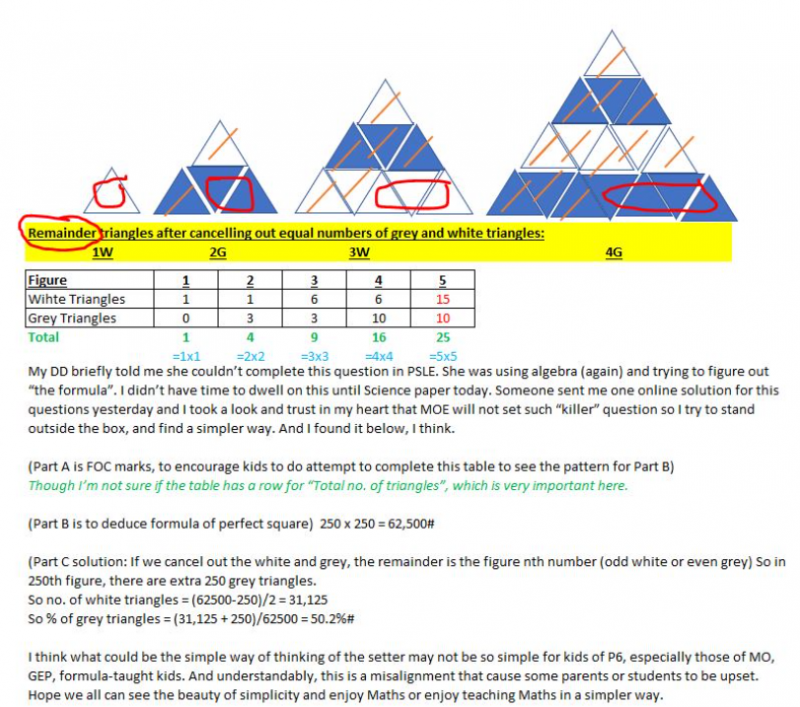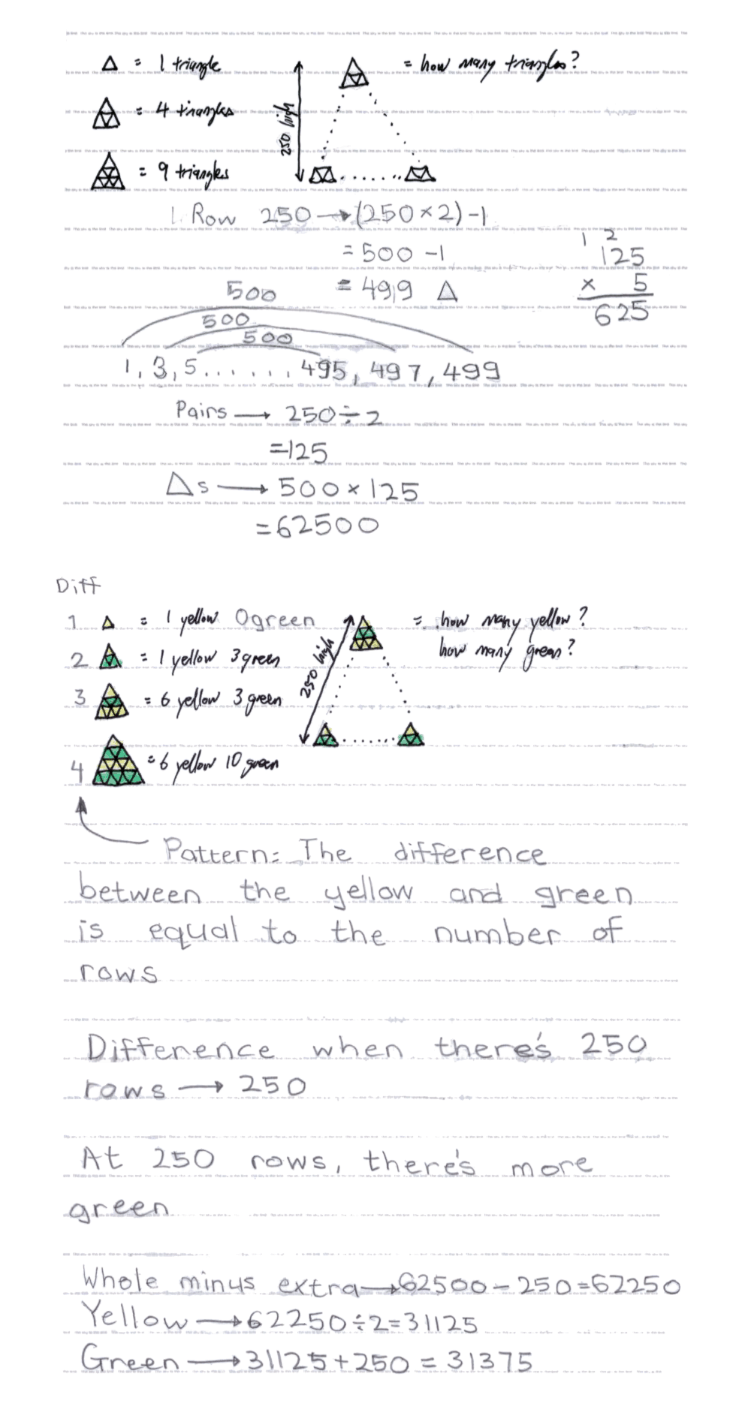# QuestionMy DD briefly told me she couldn’t complete this question in PSLE. She was using algebra (again) and trying to figure out “the formula”. I didn’t have time to dwell on this until Science paper today. Someone sent me one online solution for this questions yesterday and I took a look and trust in my heart that MOE will not set such “killer” question so I try to stand outside the box, and find a simpler way. And I found it below, I think.

(Part A is FOC marks, to encourage kids to do attempt to complete this table to see the pattern for Part B) Though I’m not sure if the table has a row for “Total no. of triangles”, which is very important here.

(Part B is to deduce formula of perfect square)  250 x 250 = 62,500#

(Part C solution: If we cancel out the white and grey, the remainder is the figure nth number (odd white or even grey) So in 250th figure, there are extra 250 grey triangles.

So no. of white triangles = (62500-250)/2 = 31,125

So % of grey triangles = (31,125 + 250)/62500 = 50.2%#

I think what could be the simple way of thinking of the setter may not be so simple for kids of P6, especially those of MO, GEP, formula-taught kids. And understandably, this is a misalignment that cause some parents or students to be upset. Hope we all can see the beauty of simplicity and enjoy Maths or enjoy teaching Maths in a simpler way.

These lessons are very useful, I think it is better to keep a good mind, by playing

0 Replies 0 Likes

The solution is quite easy to understand. Thank you for your posts.

moto x3m

0 Replies 0 Likes

Thanks for the answer. I was experiencing difficulties with my math homework and decided to try this source https://studyclerk.com/do-my-assignment where professionals are always happy to do my assignment. That’s why I always ask for help if feel a lack of expertise.

0 Replies 0 LikesSAHMom, your solution is indeed correct, and interestingly, my P3 daughter solved it in essentially the same way (click on image to see it in full resolution).  Instead of white-vs-grey, I used yellow-vs-green (being the colours of the highlighters I had on hand) just for better visibility.  It does not change the question or the difficulty of the question.

The crux & challenge of this question is Part (b), so I’ll focus my description on this.

When I first saw her answer, I thought she had done it wrongly, as it looked quite different from the “model answer” found online.  Then I took a closer look and realized that her answer was correct!  Surprisingly, her solution was much simpler and more elegant …and she informed me she had been solving this kind of question “all the time in P3”!  The last line did not mean that she had done this specific question or a question of similar difficulty before.  What she actually meant was that she solved it using techniques that she was already familiar with – in other words, simple lower Primary math, not upper Primary math, and certainly not algebra (which was what most adults I asked immediately tried to use).  (Such is the power of not scaring them by prefacing things with “This is very hard!  You can’t do it.”)

Now, her solution took multiple small steps, which I’ll explain in a long-winded “grown up” way for clarity.

Finding 1 – Pattern for Number of Triangles per Row

From the pyramid diagrams and the table, she realized that the pattern (what adults called “the formula”) is that the Nth row has N x 2 – 1 triangles.  For instance, the 1st row has 1 x 2 – 1 = 1 triangle, the 2nd row has 2 x 2 – 1 = 3 triangles, the 5th row has 5 x 2 – 1 = 9.  Of course, she didn’t call it a formula.  To her, it was merely a pattern.

For a pyramid with 250 rows, the 250th row (i.e. last row) has 250 x 2 – 1 = 499 triangles.

Finding 2 – Total Number of Triangles

She then used a common technique for figuring out the total number of triangles for a pyramid with 250 rows.

She paired the first row with the last row for a total of 1 + 499 = 500 triangles.  The second row & the second last row is also 500 triangles and so forth.  Since the pyramid has 250 rows, hence there are 125 pairs of 500 triangles.  This works out to 125 rows x 500 = 62,500 triangles total.

Finding 3 – Pattern in Yellow-vs-Green Triangles

First, from the table, she noticed that the difference in the number of yellow-vs-green triangles for a pyramid with N rows is always equal to N.  A pyramid with 3 rows means that the difference between yellow & green is 3.  A pyramid with 4 rows means that the difference between yellow & green is 4; and so forth. (This is the same as your solution, SAHMom).

Next, again from the table, she noticed that for pyramids with odd number of rows has more yellows, while the pyramids with even number of rows has more greens.

Combining Findings to Get the Solution

Hence, combining all the findings above, for a pyramid with 250 rows, there are 62,500 triangles (as per Finding 2 above) and there are 250 more greens (as per Finding 3 above).

The rest of the math is easy (or as she perceives it – a standard “Primary 3” question).  Since there are 250 more greens, the total minus this “extra” then divided by two gives the number of yellow triangles.  This result plus the 250 extra gives the number of green triangles.  Done!

Conclusion

Same as you, SAHMom, I did not believe that the “model answer” online was actually the real “model answer”.  The pattern was just too complex.  The above solution is solved elegantly using fairly “easy” math patterns & operations.

0 Replies 0 Likes

a)
Method 1 :
1 + 5 + 9 = 15 (white triangles)
3 + 7 = 10 (grey triangles)

Method 2 :
5 x 5 = 25
25 – 5 = 20
20/2 = 10 (grey triangles)
10 + 5 = 15 (white triangles)

b)
250 x 250 = 62500 (grey and white triangles)

c)
250 x 250 = 62500
62500 – 250 = 62250
62250/2 = 31125 (white triangles)
31125 + 250 = 31375 (grey triangles)
(31375/62500) x100% = 50.2%

Ans : a) 15 (white), 10 (grey); b) 62500 (grey and white) and c) 50.2%

0 Replies 0 Likes